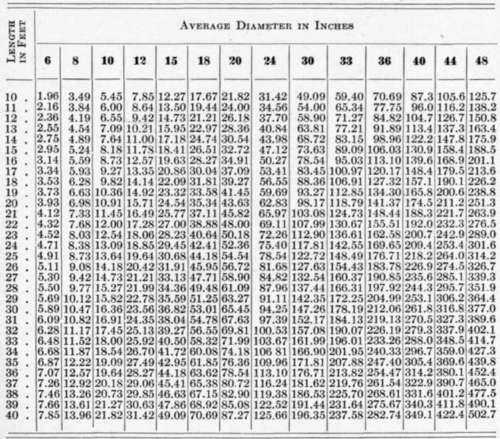This method is very simple, because it requires only two measurements of the log — the diameter at the middle and the length. Tables showing the areas of circles in these units are readily accessible, and also tables showing the cubic contents of logs of different middle diameters and lengths, so that there is no computation necessary.

Method of cubing logs by measurement of the length and end diameters.

By this method the diameters of the two ends of the log and its length are measured. The volume is obtained by multiplying the average of the areas of circles that correspond to end diameters by the length: —in which V is the volume of the log in cubic feet, B and b are the areas in square feet that correspond to the diameters of the two ends, and L is the length in feet.

Example: A log is 12 feet long, and the diameters at the ends are 16 and 18 inches. The areas that correspond to the end diameters are found in a table of circular areas, and used in the formula, as follows: —This method requires one more measurement than the previous, and is therefore not as rapid for ordinary work in commercial scaling. It is, however, a very convenient formula for determining the contents of logs where it is not possible to take the measurement at the middle, as on logs piled on a skidway.

Solid cubic contents of logs (in cubic feet)Cubic Contents of Square Timber in Round Logs (Woodsman's

Handbook)

The most common methods of determining the cubic contents of square timber that may be cut from round logs is the so-called Two-thirds Rule, and the Inscribed Square Rule.

The two-thirds rule.

In the Two-thirds Rule the diameter of the log is taken at its middle point, or the diameters of the two ends of the log are averaged. The diameter of the log is reduced one-third to allow for slab, and the remaining two-thirds is taken as the width of the square piece which may be hewed or sawed out of the log. The cubic contents of the squared log are then obtained by squaring this width and multiplying by the length of the log.

Square timber cut from round logs (in cubic feet) (Inscribed-Square Rule)The inscribed-square rule.

The Inscribed-Square Rule gives the cubic contents of square pieces which can be exactly inscribed in cylinders of different sizes. The width of this square piece is usually obtained by multiplying the diameter of the cylinder by 17 and dividing the result by 24, or by multiplying the diameter by 0.7071. This rule of thumb for calculating the width of the inscribed square piece is based on the fact that one side of the square inscribed in a circle 24 inches in diameter is 17 inches long.

The exact mathematical rule for determining the side of a square inscribed in a circle is to square the diameter, divide by 2, and extract the square root. The table on the preceding page was computed by this method.

Practically the same results are obtained by the Seventeen-inch Rule, which is based on the fact that a 17-inch log will square 12 inches. According to the Seventeen-inch Rule, the cubic contents of a log are obtained as follows: Multiply the square of the diameter of the log by its length, and divide by the square of 17.